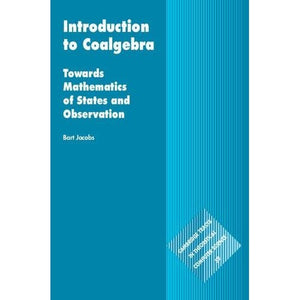># Introduction to Coalgebra: Towards Mathematics of States and Observation (Cambridge Tracts in Theoretical Computer Science)

• £65.99
• Save £60

Bart Jacobs
Cambridge University Press, 10/27/2016
EAN 9781107177895, ISBN10: 1107177898

Hardcover, 494 pages, 23.5 x 15.9 x 3.2 cm
Language: English

The area of coalgebra has emerged within theoretical computer science with a unifying claim: to be the mathematics of computational dynamics. It combines ideas from the theory of dynamical systems and from the theory of state-based computation. Although still in its infancy, it is an active area of research that generates wide interest. Written by one of the founders of the field, this book acts as the first mature and accessible introduction to coalgebra. It provides clear mathematical explanations, with many examples and exercises involving deterministic and non-deterministic automata, transition systems, streams, Markov chains and weighted automata. The theory is expressed in the language of category theory, which provides the right abstraction to make the similarity and duality between algebra and coalgebra explicit, and which the reader is introduced to in a hands-on manner. The book will be useful to mathematicians and (theoretical) computer scientists and will also be of interest to mathematical physicists, biologists and economists.

1. Motivation
2. Coalgebras of polynomial functors
3. Bisimulations
4. Logic, lifting and finality